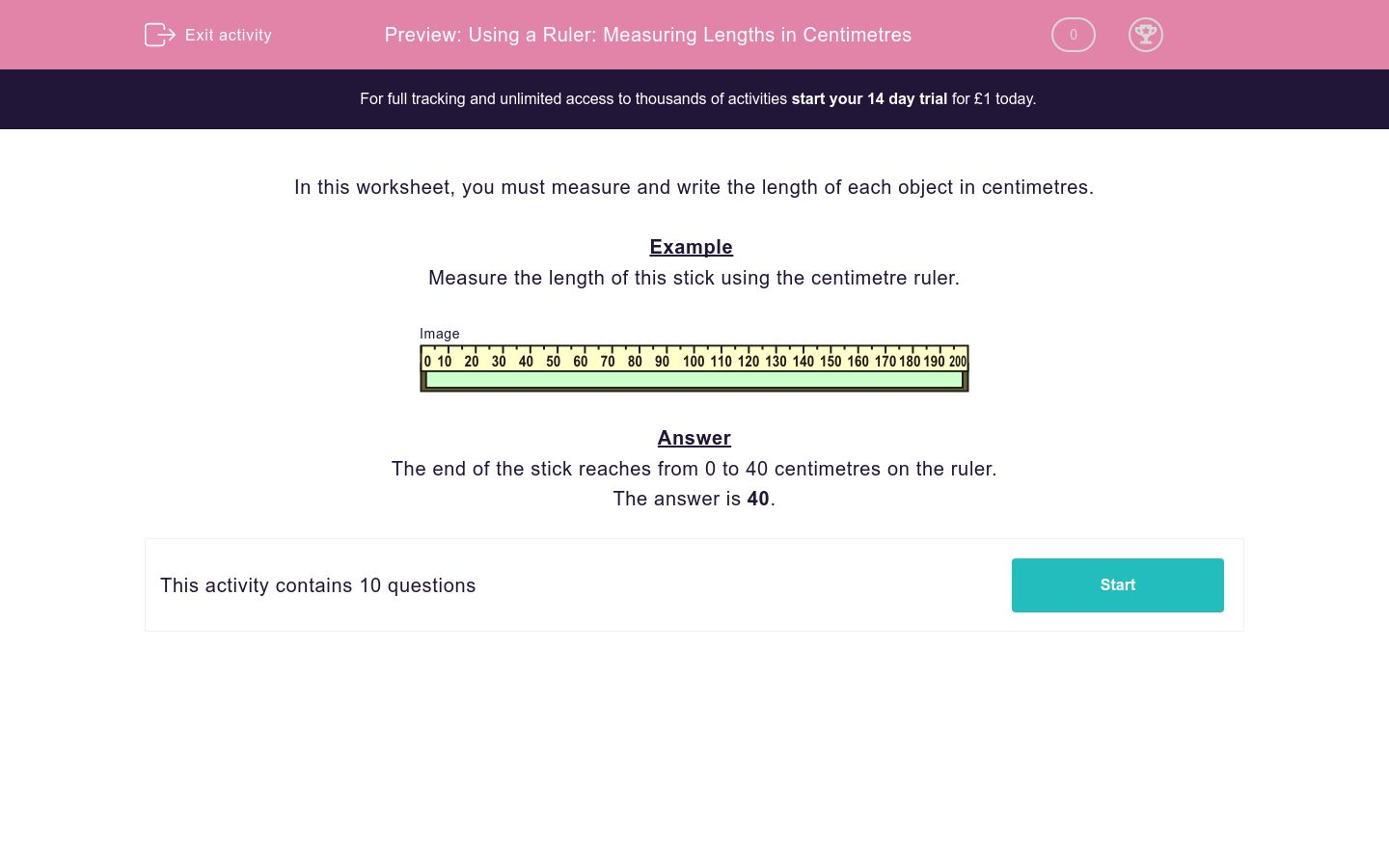# Using a Ruler: Measuring Lengths in Centimetres

In this worksheet, students measure a length in centimetres by reading a scale on a ruler.Key stage:  KS 2

Curriculum topic:   Maths and Numerical Reasoning

Difficulty level:### QUESTION 1 of 10

In this worksheet, you must measure and write the length of each object in centimetres.

Example

Measure the length of this stick using the centimetre ruler.The end of the stick reaches from 0 to 40 centimetres on the ruler.

Measure the length of this sign using this centimetre ruler.

Just write the number.Measure the length of this doll house using this centimetre ruler.

Just write the number.Measure the length of this stick using this centimetre ruler.

Just write the number.Measure the length of this doll house using this centimetre ruler.

Just write the number.Measure the length of this stick using this centimetre ruler.

Just write the number.Measure the length of this fence using this centimetre ruler.

Just write the number.Measure the length of this stick using this centimetre ruler.

Just write the number.Measure the length of this fence using this centimetre ruler.

Just write the number.Measure the length of this stick using this centimetre ruler.

Just write the number.Measure the length of this fence using this centimetre ruler.

Just write the number.• Question 1

Measure the length of this sign using this centimetre ruler.

Just write the number.10
• Question 2

Measure the length of this doll house using this centimetre ruler.

Just write the number.30
• Question 3

Measure the length of this stick using this centimetre ruler.

Just write the number.30
• Question 4

Measure the length of this doll house using this centimetre ruler.

Just write the number.50
• Question 5

Measure the length of this stick using this centimetre ruler.

Just write the number.60
• Question 6

Measure the length of this fence using this centimetre ruler.

Just write the number.40
• Question 7

Measure the length of this stick using this centimetre ruler.

Just write the number.70
• Question 8

Measure the length of this fence using this centimetre ruler.

Just write the number.80
• Question 9

Measure the length of this stick using this centimetre ruler.

Just write the number.120
• Question 10

Measure the length of this fence using this centimetre ruler.

Just write the number.90
---- OR ----

Sign up for a £1 trial so you can track and measure your child's progress on this activity.

### What is EdPlace?

We're your National Curriculum aligned online education content provider helping each child succeed in English, maths and science from year 1 to GCSE. With an EdPlace account you’ll be able to track and measure progress, helping each child achieve their best. We build confidence and attainment by personalising each child’s learning at a level that suits them.

Get started